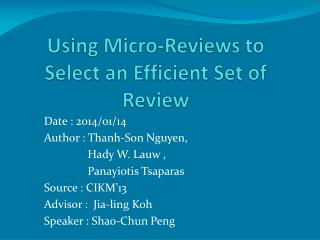DownloadDownload PresentationUsing Micro-Reviews to Select an Efficient Set of Review

Using Micro-Reviews to Select an Efficient Set of Review

Download PresentationUsing Micro-Reviews to Select an Efficient Set of Review

- - - - - - - - - - - - - - - - - - - - - - - - - - - E N D - - - - - - - - - - - - - - - - - - - - - - - - - - -
Presentation Transcript

1. Using Micro-Reviews to Select an Efficient Set of Review Date : 2014/01/14 Author : Thanh-Son Nguyen, Hady W. Lauw , Panayiotis Tsaparas Source : CIKM’13 Advisor : Jia-ling Koh Speaker : Shao-Chun Peng

2. Outline • Introduction • Method • Experiments • Conclusion

3. Introduction • Motivation • We can find ample review in Web sources, but which are better to show the experiences of customer

4. Introduction • Reviews: • unstructured, lengthy, verbose • From Web(e.g. Yelp, Amazon.com) • Micro-reviews(tips): • short, concise, focused • From micro-blogging (e.g. Facebook, Twitter)

5. Purpose • Bring together these two diverse type of review content, to obtain something that is more than the sum of its part

6. Framework pairs Reviews Algorithm EffMaxCover • Matching review and tip • Syntactically • Semantic • Sentiment tips output α β Set of reviews k

7. Outline • Introduction • Method • Experiments • Conclusion

8. Syntactically similarity • A review sentence and a tip are syntactically similar if they share important keywords • SynSim(s; t) = cosine(s; t) • Compute s and t with standard tf-idf in corpus tf-idf a:0.5 b:0.3 c:0.7 d:0.1 e:0.3 f:0.2 s:abcd Vector s={0.5, 0.3, 0.7, 0.1, 0,0} Vectort={0, 0, 0.7, 0.1, 0.3, 0.2} t:cdef

9. Semantic similarity • A review sentence and a tip are semantically similar, when they are describing the same concept • SemSim(s; t) = JSD(θs; θ t) • θs , θt : probability distribution over the topics • JSD:compute probability similarity pre-process: LDA classify

10. Sentiment similarity • A matching pair of review sentence and tip should also represent the same sentiment • SentSim(s; t) = polarity(s)× polarity(t) • maximum entropy classier (MEM) • P(C+|d)+P(C-|d) = 1 [0,1] • polarity(s)= 2P(C+|d)-1 [-1,1]

11. Matching Function • Binary classification framework • Syntactically similarity • Semantic similarity • Sentiment similarity

12. Coverage • Review: R • Tips: T • Sentence :s Coverage(R)=|TR|

13. Efficiency • Review: R • Tips: T • Sentence: s

14. Merger Coverage • Cov(S)=|URЄS TR| • Normalized: Cov(S)/|T|

15. Merger Efficiency • More involve than Coverage • Minimum • Average • Bag • View a collection S as a single review consisting of the union of sentences

16. EffMaxCover • Bi-criterion optimization problem • NP-hard • Constraining efficiency , max coverage • Eff(S)>=α find the max coverage

17. Algorithm • Greedy approach • Gain(R)=Cov(S U R) - Cov(S) • Cost(R)=β(1-EFF(R))+(1- β) • For each round add the max Gain(R)/Cost(R) to the set

18. Outline • Introduction • Method • Experiments • Conclusion

19. Data set • March 2012 • 110 restaurant in New York (delete tip<=50) • 102 restaurant • 96612 reviews (584~3460) • 14740 tips (51~498)

20. Experiments

21. Experiments β is an effective way to gain eff with min loss in cov

22. Experiments

23. Outline • Introduction • Method • Experiments • Conclusion

24. Conclusion • Use micro-review for finding an efficient review set • Design EffMaxCover to the selection problem